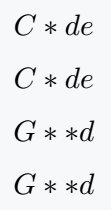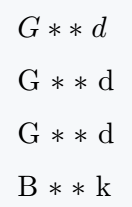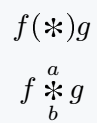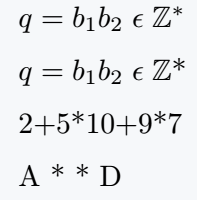# How do you type an asterisk(＊) in LaTeX?

The Asterisk symbol is denoted by the command \ast.  Same symbol is on your keyboard, but it has two different uses.

Symbol Asterisk
Type of symbol Mathematics
Package No
Argument No
Latex command \ast
Example \ast → ＊
\documentclass{article}
\begin{document}
$C\ast de$
$C*de$
$G\ast\ast d$
$G**d$
\end{document}

Output :At the same time, we use two asterisk symbols in pairs in different words. If you look at the output above you will understand that there is a space-related problem.

Which is shown in the following code.

\documentclass{article}
\usepackage{MnSymbol}
\begin{document}
$G\ast\ast\; d$\$6pt] G \ast\;\ast d \\[6pt] G *\;* d \\[6pt] B *\;* k \end{document} Output :## Big asterisk symbol in LaTeX \bigast command is not the default. For this, you need to take the help of mathabx package. \documentclass{article} \usepackage{mathabx} \begin{document} \[ f(\bigast)g$
$f\bigast_b^a g$
\end{document}

Output :## Use asterisk symbol in Superscript in LaTeX

If you use this symbol from the keyboard in direct text mode, the asterisk symbol will act as a superscript.

And you will need to manually complete the same task using the command.

\documentclass{article}
\usepackage{amsfonts}
\begin{document}
$q=b_1b_2\; \epsilon\; \mathbb{Z}^\ast$ \\[6pt]
$q=b_1b_2\; \epsilon\; \mathbb{Z}$* \\[6pt]
2+5*10+9*7
A * * D
\end{document}

Output :## Asterisk symbols of different styles

Below are a variety of styled asterisk symbols represented in a latex document using the bbding and pifont packages.

\documentclass{article}
\usepackage{bbding,pifont}
\begin{document}
% Use bbding package
\SixFlowerOpenCenter, \SixFlowerPetalRemoved, \Asterisk\\[6pt]
\AsteriskBold, \AsteriskCenterOpen, \AsteriskRoundedEnds \\[6pt]
\AsteriskThin, \AsteriskThinCenterOpen, \SixFlowerRemovedOpenPetal\\[6pt]
% Use pifont package
\ding{81}, \ding{82}, \ding{83}\\[6pt]
\ding{91}, \ding{92}, \ding{93}\\[6pt]
\end{document}

Output :#### Md Jidan Mondal

LaTeX expert with over 10 years of experience in document preparation and typesetting. Specializes in creating professional documents, reports, and presentations using LaTeX.